TitleBeginning AlgebraLearning Objectives

 After completing this tutorial, you should be able to: Read a bar graph. Read a line graph. Read a double line graph. Draw and read a Venn diagram.Introduction

 In this tutorial we will be reading graphs.  Graphs can be used to visually represent the relationship of data.  It can help organize and show people statistics, which can be good for some and not so good for others, depending on what the statistics show.  Organizing data graphically can come in handy in fields like business, sports, teaching, politics, advertising, etc..  Let's start looking at some graphs.Tutorial

 Bar Graph

A bar graph can be used to give a visual representation of the relationship of data that has been collected.

It is made up of a vertical and a horizontal axis and bars that can run vertically or horizontally.

 Vertical Bar Graph

If the bars are vertical, match the top of the bar with the vertical axis found at the side of the overall graph to find the information the bar associates with on the vertical axis.  You will find what the bar associates with on the horizontal axis at the base of the bar.

The bar graph below has vertical bars: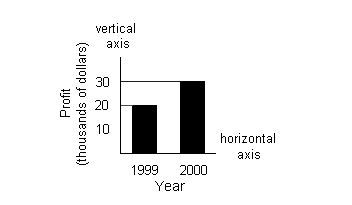The horizontal axis represents years and the vertical axis represents profit in thousands of dollars.  The first bar on the left associates with the year 1999 AND the profit of \$20,000.  The red line shows how the top of the bar lines up with 20 on the vertical axis.  The second bar from the left associates with the year 2000 and the profit of \$30,000.  The blue line shows how the top of the bar lines up with 30 on the vertical axis.

 Horizontal Bar Graph

If the bars are horizontal, match the right end of the bar with the horizontal axis found at the bottom of the overall graph to find the information the bar associates with on the horizontal axis.  You will find what the the bar associates with on the vertical axis at the left end of the bar.

The bar graph below has horizontal bars:

(Note that this graph shows the same information the above graph does, just with horizontal bars instead of vertical bars.)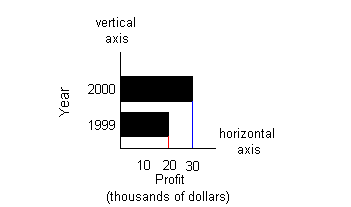The vertical axis represents years and the horizontal axis represents profit in thousands of dollars.  The first bar on the bottom associates with the year 1999 AND the profit of \$20,000.  The red line shows how the right end of the bar lines up with 20 on the horizontal axis.  The second bar from the bottom associates with the year 2000 and the profit of \$30,000.  The blue line shows how the right end of the bar lines up with 30 on the horizontal axis.Example 1:  The bar graph below shows the number of students in a math class that received the grades shown.  Use this graph to answer questions 1a - 1d. 1a.   Find the number of students who received an A. 1b.  Find the number of students who received an F. 1c.  Find the number of students who passed the course (D or higher). 1d.  Which grade did the most students receive?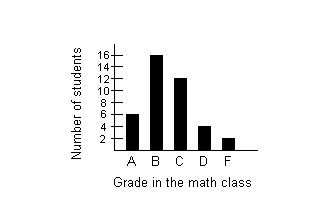The bar that associates with the grade A is the first bar on the left.  The top of that bar matches with 6 on the vertical axis. 6 students received an A.

 The bar that associates with the grade F is the fifth bar from the left.  The top of that bar matches with 2 on the vertical axis. 2 students received an F.

 1c.  Find the number of students who passed the course (D or higher). (return to bar graph)

 We will have to do a little calculating here.  We will need to find the number of students that received an A, B C, and D and then ad them together. The bar that associates with the grade A is the first bar on the left.  The top of that bar matches with 6 on the vertical axis. The bar that associates with the grade B is the second bar from the left.  The top of that bar matches with 16 on the vertical axis. The bar that associates with the grade C is the third bar from the left.  The top of that bar matches with 12 on the vertical axis. The bar that associates with the grade D is the fourth bar from the left.  The top of that bar matches with 4 on the vertical axis.   6 + 16 + 12 + 4 = 38 students passed the course.

 It looks like more students received a B than any other single grade.Example 2:  The bar graph below shows the number of civilians holding various federal government jobs.   Use the graph to answer questions 2a - 2d. 2a.  About how many civilians work for Congress? 2b.  About how many civilians work for the State Department? 2c.  About how many civilians work for the armed forces (Navy, Air Force, and Army)? 2d.  Which federal government job listed has the most civilian workers?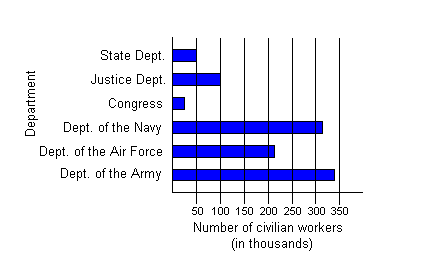The bar that associates with Congress is the fourth bar up.  The right of that bar lines up a little to the left of 50 on the horizontal axis.  Note how the question asks ABOUT how many.  In some cases, if it does not directly line up with a number that is marked you may need to approximate.  This is very close to and less than 50. A good approximation is 25. About 25,000 civilians work for Congress.

 The bar that associates with the State Department is the sixth bar up.  The right of that bar lines up with 50 on the horizontal axis.  About 50,000 civilians work for the State Department.

 2c.  About how many civilians work for the armed forces (Navy, Air Force, and Army)? (return to bar graph)

 We will have to do a little calculating on this one.  We will need to find the number of civilians that work for each branch of the armed services and then add them up. The bar that associates with the Navy is the third bar up.  The right of that bar ends between 300 and 350 on the horizontal axis. 310 is a good approximation for this number. The bar that associates with the Air Force is the second bar up.  The right of that bar ends between 200 and 250 on the horizontal axis. 210 is a good approximation for this number. The bar that associates with the Army is the first bar from the bottom.  The right of that bar ends just under 350 on the horizontal axis. 340 is a good approximation for this number. About 310,000 + 210,000 + 340,000 = 860,000 civilians work for the State Department.

 2d.  Which federal government job listed has the most civilian workers? (return to bar graph)

 It looks like the Army has the most civilian workers.

 Line Graph

A line graph is another way to give a visual representation of the relationship of data that has been collected.

It is made up of a vertical and horizontal axis and a series of points that are connected by a line.

Each point on the line matches up with a corresponding vertical axis and horizontal axis value on the graph.

In some cases, you are giving a value from the horizontal axis and you need to find its corresponding value from the vertical axis.  You find the point on the line that matches the given value from the horizontal axis and then match it up with its corresponding vertical axis value to find the value you are looking for.  You would do the same type of process if you were given a vertical axis value and needed to find a horizontal axis value.

The graph below is a line graph:

(Note that this graph shows the same information the above graphs under vertical and horizontal graphs do, just with a line instead of bars.)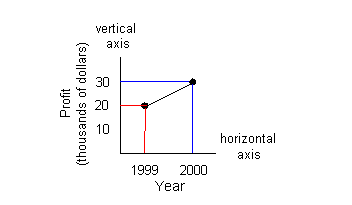The horizontal axis represents years and the vertical axis represents profit in thousands of dollars.  The first point on the left associates with the year 1999 AND the profit of \$20,000.  The red line shows how it lines up with 20 on the vertical axis and 1999 on the horizontal axis.  The second point from the left associates with the year 2000 and the profit of \$30,000.  The blue line shows how it lines up with 30 on the vertical axis and 2000 on the horizontal axis.Example 3:  The line graph below shows the distance traveled of a vacationer going 70 mph down I-40 from 0 to 4 hours.   Use the graph to answer questions 3a - 3b. 3a.  How far has the vacationer traveled at 3 hours? 3b.  How long does it take the vacationer to travel 140 miles?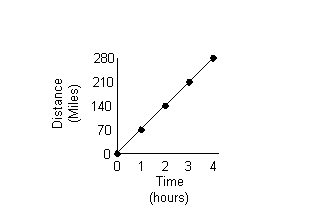3a.  How far has the vacationer traveled at 3 hours? (return to line graph)

 The point that matches with 3 on the horizontal axis also matches with 210 on the vertical axis. The vacationer has traveled 210 miles.

 3b.  How long does it take the vacationer to travel 140 miles? (return to line graph)

 The point that matches with 140 on the vertical axis also matches with 2 on the horizontal axis. It takes the  vacationer 2 hours to travel 140 miles.Example 4:  The line graph below shows the profit a local candy company made over the months of September through December of last year.   Use the graph to answer questions 4a - 4c. 4a.  About how much was the profit in the month of October? 4b.  Which month had the lowest profit? 4c.  What is the difference between the profits of November and December?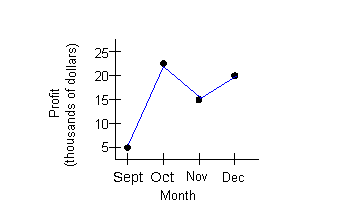The point that matches with October on the horizontal axis also matches between 20 and 25 on the vertical axis.  It looks to be about 23. The profit for the month of October is about \$23,000.

 It looks like September had the lowest profit.

 4c.  What is the difference between the profits of November and December? (return to line graph)

 The point that matches with November on the horizontal axis also matches with 15 on the vertical axis.  The point that matches with December on the horizontal axis also matches with 20 on the vertical axis.  The difference between the profits of November and December would be 20,000 - 15,000 = \$5,000.

 Double Line Graph

A double line graph is another way to give a visual representation of the relationship of data that has been collected.

It is similar to the line graph mentioned above.  The difference is there are two lines of data instead of one.

It is made up of a vertical and horizontal axis and two series of points each one connected by a line.

The legend will show which line represents what set of points.  Most times a solid line and a dashed line are used.  But varying colors can also distinguish the two lines apart.

Each point on each line matches up with a corresponding vertical axis and horizontal axis value on the graph.

In some cases, you are giving a value from the horizontal axis and you need to find its corresponding value from the vertical axis.  You find the point on the line that matches the given value from the horizontal axis and then match it up with its corresponding vertical axis value to find the value you are looking for.  You would do the same type of process if you were given a vertical axis value and needed to find a horizontal axis value.

The graph below is a double line graph:The horizontal axis represents the year and the vertical axis represents profit in thousands of dollars.  The legend towards the top of the graph indicates which line represents which product.  The solid line corresponds with Product A and the dashed line goes with Product B. The first point on the solid line on the left associates with the year 1995 AND the profit of \$30,000. The second point on the solid line from the left associates with the year 1996 AND the profit of \$40,000. The third point on the solid line from the left associates with the year 1997 AND the profit of \$40,000. The fourth point on the solid line from the left associates with the year 1998 AND the profit of \$30,000. The fifth point on the solid line from the left associates with the year 1999 AND the profit of \$60,000.   The first point on the dashed line on the left associates with the year 1995 AND the profit of \$20,000. The second point on the dashed line from the left associates with the year 1996 AND the profit of \$20,000. The third point on the dashed line from the left associates with the year 1997 AND the profit of \$15,000. The fourth point on the dashed line from the left associates with the year 1998 AND the profit of \$40,000. The fifth point on the dashed line from the left associates with the year 1999 AND the profit of \$50,000.Example 5:  The double line graph below shows the total enrollment of students in a local college from 1990 - 1995, broken down into part-time and full-time students.  Use the graph to answer questions 5a - 5c. 5a.  What was the full-time enrollment in 1992? 5b.  For what year shown on the graph did the number of part-time students exceed the previous year’s number of part-time students by the greatest number? 5c.  What was the total enrollment from 1993 to 1995?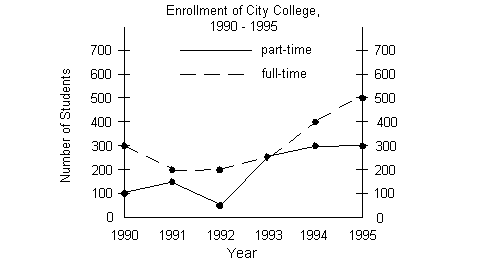5a.  What was the full-time enrollment in 1992? (return to double line graph)

 Since we are looking for full-time students, are we going to look at the solid or dashed line? According to the legend, we need to look at the dashed line.  The point that is on the dashed line and  matches with 1992 on the horizontal line also matches with 200 on the vertical line. There were 200 full-time students enrolled in 1992.

 5b.  For what year shown on the graph did the number of part-time students exceed the previous year’s number of part-time students by the greatest number? (return to double line graph)

 Since we are looking for part-time students, are we going to look at the solid or dashed line? According to the legend, we need to look at the solid line.  When looking at the graph, we are only interested in a rise in the number of part-time students.  From 1990 to 1991, the number of part-time students went up 100 to 150.  From 1991 to 1992, it went down from 150 to 50.  From 1992 to 1993, there was increase from 50 to 250.  From 1993 to 1994, there was another increase, this time from 250 to 300.  The last years, 1994 - 1995, it held steady at 300.  So, what year exceeded the previous number of part-time students by the greatest number? Looks like 1993.  There were 200 more part-time students in 1993 than there were in 1992.

 5c.  What was the total enrollment from 1993 to 1995? (return to double line graph)

 Let’s break this down into part-time and full-time students.  Looking at the dashed line to see the number of full-time students we get 250 + 400 + 500 = 1150. Looking at the solid line to see the number of part-time students we get 250 + 300 + 300 = 850. Putting those together, we have 1150 + 850 = 2000 students who were enrolled from 1993 to 1995.

 Venn Diagrams

 Venn diagrams are a visual way of organizing information.  It can be very helpful when you have a problem to solve that categorizes or shows relationships between things.  A common use for Venn diagrams is analyzing the results of a survey.  For example, you may have a survey of students asking them which classes they like and perhaps you listed math and english.  The student could check 0, 1, or 2 of these choices.  You would strategically place the results in a Venn diagram.  If they only choose math, then they would go in a particular  region of the diagram that shows that, if they picked both, they would go into the area of the diagram that depicts that, etc.  Of course there are other uses for the Venn diagram, that is one of the more common ones. The graph below is a Venn diagram: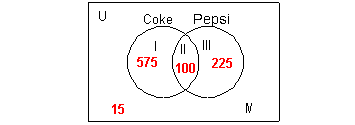This diagram represents the results of a survey of people who were asked if they liked Coke or Pepsi.  They could choose only Coke, only Pepsi, both, or neither. Note that a lot of times you do not see the letter U or the roman numerals on a Venn Diagram (just the box and the circles), I use them as references so you know what area of the diagram I'm talking about in the lesson.  The rectangle box represents the universal set U.  The universal set  is the set of all elements considered in a problem.  In this example, the universal set are all the people who took the survey.  The circles represent the categories or subsets involving the universal set.  In this example, the two categories or choices on the survey were Coke and Pepsi.  When you draw a Venn diagram, you want to overlap the circles in case there are some that pick both categories.  We need to make sure we accurately place those people and do not count them more than one time. The roman numerals are called region numbers.   Region I represents everyone who selected ONLY Coke which was 575 people. Region II is where the two circles intersect or overlap.  It represents everyone who selected BOTH Coke and Pepsi which was 100 people. Region III represents everyone who selected ONLY Pepsi which was 225 people. Region IV is inside the rectangle, but outside the circles.  It  represents everyone who selected NEITHER Coke nor Pepsi which was 15 people.Example 6:  A teacher took a survey on pets in her class of 40 students.  12 students said they had a cat.  9 students said they had a dog.  2 said they had both a cat and a dog.  How many students picked neither?  How many students had only a cat?  How many students had only a dog?

 The first thing we need to do is draw a Venn diagram with two adjoining circles - one for cats and one for dogs.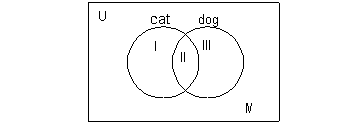Now we need to fill in numbers into the correct regions based on the information that was given. We need to start with something that only goes with one region and then work our way out from that.  The only statement that deals with one region is 2 said they had both a cat and a dog.  That would correlate with region II.  So in region II, we would put a 2 as shown below: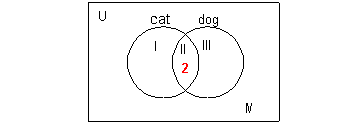Next let's look at the statement 12 students said they had a cat.  Be careful here.  It is very tempting to put a 12 in region I - but region I is reserved for those students who ONLY have a cat, which is different.  When it says they had a cat, it means they checked it off on the survey and may or may not have checked off dog also.  The cat circle includes regions I and II.   Since we already have region II filled in with a 2, we can use that with the fact that I and II have to add up to be 12 to figure out what goes in region I - what do you think??? If we take 12  - 2 we get 10 left that have no other place to go but region I. This puts everybody in the correct spot AND does not count students more than 1 time. We can use the same type of argument when working with the statement "9 students said they had a dog."  Again, it did not say ONLY dog - so 9 will have to fit into regions II and III and since we already have II filled in with 2 students that will leave 9 - 2 = 7 to go into region III.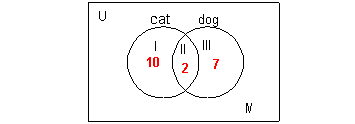That leaves us with having to fill in region IV. We can use the fact that 40 students answered the survey and that we have three of the four regions filled in.  So we can take the total of 40 and subtract everyone that is already accounted for and that will leave us who is in region IV:  40 - 10 - 2 - 7 = 21.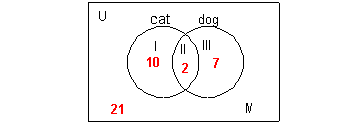Overall, you need to start with the information that goes with only one region first.  If you start with something that goes with more than one part, then you will not know how to split it up appropriately so everyone is in the right spot AND is not counted more than one time.  For example, if we would have started with the fact that there were 40 students, we would have had trouble because all four regions would make up all the students surveyed.  We wouldn't know how to appropriately split that 40 up.  Or if we looked at the fact that 12 choose a cat to start with, we would not know how to split it between the two regions that make up the cat circle.   Final answer: Looking at the Venn diagram, the students that chose neither would be in region IV, which comes out to be 21. The students who chose only a cat would be in region I, which is 10.  The students who chose only a dog would be in region III, which is 7.Practice Problems

 These are practice problems to help bring you to the next level.  It will allow you to check and see if you have an understanding of these types of problems. Math works just like anything else, if you want to get good at it, then you need to practice it.  Even the best athletes and musicians had help along the way and lots of practice, practice, practice, to get good at their sport or instrument.  In fact there is no such thing as too much practice. To get the most out of these, you should work the problem out on your own and then check your answer by clicking on the link for the answer/discussion for that  problem.  At the link you will find the answer as well as any steps that went into finding that answer.Practice Problems 1a - 1c: The bar graph below shows the profit a cd store made over the months of September through December of last year.   Use the graph to answer questions 1a - 1c.1a.  About how much was the profit in September? (answer/discussion to 1a - 1c)

 1b.  Which month had the highest profit? (answer/discussion to 1a - 1c)

 1c.  What is the difference between the profits of October and November? (answer/discussion to 1a - 1c)Practice Problems 2a - 2c: The line graph below shows last week's high temperatures in Fahrenheit.   Use the graph to answer questions 2a - 2c.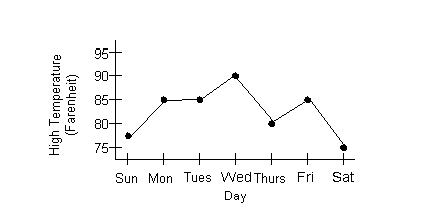2a.  How much was Thursday's high temperature? (answer/discussion to 2a - 2c)

 2b.  Which day had the lowest high temperature? (answer/discussion to 2a - 2c)

 2c.  What temperature occurred the most? (answer/discussion to 2a - 2c)Practice Problems 3a - 3c: The double line graph below shows the total enrollment of people who work out at a local gym from 1998 - 2002, broken down into males and females.  Use the graph to answer questions 3a - 3c.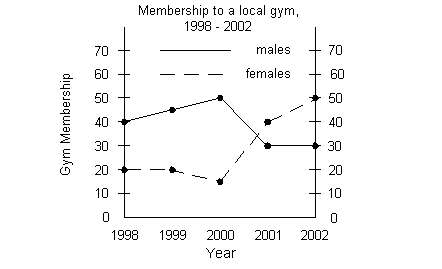3a.  What was the ratio between male gym members and female gym members in 2000? (answer/discussion to 3a - 3c)

 3b.  For what year shown on the graph did male gym membership not change from the year before? (answer/discussion to 3a - 3c)

 3c.  What was the total enrollment of the gym from 1998 to 2000? (answer/discussion to 3a - 3c)Practice Problems 4a - 4c: A group of students were asked if they liked rock or country music.  The results were as follows: 27 said they liked rock, 20 said they liked country, 5 liked both, and 3 liked neither.

 4a.  How many students chose only rock? (answer/discussion to 4a - 4c)

 4b.  How many students chose only country? (answer/discussion to 4a - 4c)

 4c.  How many students were surveyed? (answer/discussion to 4a - 4c)Need Extra Help on these Topics?

 Go to Get Help Outside the Classroom found in Tutorial 1: How to Succeed in a Math Class for some more suggestions.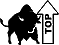Last revised on July 25, 2011 by Kim Seward.